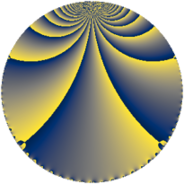# Properties

 Label 64.2.b.aLevel $64$ Weight $2$ Character orbit 64.b Analytic conductor $0.511$ Analytic rank $0$ Dimension $2$ CM discriminant -8 Inner twists $4$

# Learn more

## Newspace parameters

 Level: $$N$$ $$=$$ $$64 = 2^{6}$$ Weight: $$k$$ $$=$$ $$2$$ Character orbit: $$[\chi]$$ $$=$$ 64.b (of order $$2$$, degree $$1$$, minimal)

## Newform invariants

 Self dual: no Analytic conductor: $$0.511042572936$$ Analytic rank: $$0$$ Dimension: $$2$$ Coefficient field: $$\Q(\sqrt{-1})$$ Defining polynomial: $$x^{2} + 1$$ Coefficient ring: $$\Z[a_1, a_2, a_3]$$ Coefficient ring index: $$2$$ Twist minimal: yes Sato-Tate group: $\mathrm{U}(1)[D_{2}]$

## $q$-expansion

Coefficients of the $$q$$-expansion are expressed in terms of $$i = \sqrt{-1}$$. We also show the integral $$q$$-expansion of the trace form.

 $$f(q)$$ $$=$$ $$q + 2 i q^{3} - q^{9} +O(q^{10})$$ $$q + 2 i q^{3} - q^{9} -6 i q^{11} -6 q^{17} + 2 i q^{19} + 5 q^{25} + 4 i q^{27} + 12 q^{33} -6 q^{41} + 10 i q^{43} -7 q^{49} -12 i q^{51} -4 q^{57} -6 i q^{59} -14 i q^{67} + 2 q^{73} + 10 i q^{75} -11 q^{81} + 18 i q^{83} + 18 q^{89} + 10 q^{97} + 6 i q^{99} +O(q^{100})$$ $$\operatorname{Tr}(f)(q)$$ $$=$$ $$2q - 2q^{9} + O(q^{10})$$ $$2q - 2q^{9} - 12q^{17} + 10q^{25} + 24q^{33} - 12q^{41} - 14q^{49} - 8q^{57} + 4q^{73} - 22q^{81} + 36q^{89} + 20q^{97} + O(q^{100})$$

## Character values

We give the values of $$\chi$$ on generators for $$\left(\mathbb{Z}/64\mathbb{Z}\right)^\times$$.

 $$n$$ $$5$$ $$63$$ $$\chi(n)$$ $$-1$$ $$1$$

## Embeddings

For each embedding $$\iota_m$$ of the coefficient field, the values $$\iota_m(a_n)$$ are shown below.

For more information on an embedded modular form you can click on its label.

Label $$\iota_m(\nu)$$ $$a_{2}$$ $$a_{3}$$ $$a_{4}$$ $$a_{5}$$ $$a_{6}$$ $$a_{7}$$ $$a_{8}$$ $$a_{9}$$ $$a_{10}$$
33.1
 − 1.00000i 1.00000i
0 2.00000i 0 0 0 0 0 −1.00000 0
33.2 0 2.00000i 0 0 0 0 0 −1.00000 0
 $$n$$: e.g. 2-40 or 990-1000 Significant digits: Format: Complex embeddings Normalized embeddings Satake parameters Satake angles

## Inner twists

Char Parity Ord Mult Type
1.a even 1 1 trivial
8.d odd 2 1 CM by $$\Q(\sqrt{-2})$$
4.b odd 2 1 inner
8.b even 2 1 inner

## Twists

By twisting character orbit
Char Parity Ord Mult Type Twist Min Dim
1.a even 1 1 trivial 64.2.b.a 2
3.b odd 2 1 576.2.d.a 2
4.b odd 2 1 inner 64.2.b.a 2
5.b even 2 1 1600.2.d.a 2
5.c odd 4 1 1600.2.f.a 2
5.c odd 4 1 1600.2.f.b 2
7.b odd 2 1 3136.2.b.b 2
8.b even 2 1 inner 64.2.b.a 2
8.d odd 2 1 CM 64.2.b.a 2
12.b even 2 1 576.2.d.a 2
16.e even 4 1 256.2.a.a 1
16.e even 4 1 256.2.a.d 1
16.f odd 4 1 256.2.a.a 1
16.f odd 4 1 256.2.a.d 1
20.d odd 2 1 1600.2.d.a 2
20.e even 4 1 1600.2.f.a 2
20.e even 4 1 1600.2.f.b 2
24.f even 2 1 576.2.d.a 2
24.h odd 2 1 576.2.d.a 2
28.d even 2 1 3136.2.b.b 2
32.g even 8 4 1024.2.e.l 4
32.h odd 8 4 1024.2.e.l 4
40.e odd 2 1 1600.2.d.a 2
40.f even 2 1 1600.2.d.a 2
40.i odd 4 1 1600.2.f.a 2
40.i odd 4 1 1600.2.f.b 2
40.k even 4 1 1600.2.f.a 2
40.k even 4 1 1600.2.f.b 2
48.i odd 4 1 2304.2.a.h 1
48.i odd 4 1 2304.2.a.i 1
48.k even 4 1 2304.2.a.h 1
48.k even 4 1 2304.2.a.i 1
56.e even 2 1 3136.2.b.b 2
56.h odd 2 1 3136.2.b.b 2
80.k odd 4 1 6400.2.a.a 1
80.k odd 4 1 6400.2.a.x 1
80.q even 4 1 6400.2.a.a 1
80.q even 4 1 6400.2.a.x 1

By twisted newform orbit
Twist Min Dim Char Parity Ord Mult Type
64.2.b.a 2 1.a even 1 1 trivial
64.2.b.a 2 4.b odd 2 1 inner
64.2.b.a 2 8.b even 2 1 inner
64.2.b.a 2 8.d odd 2 1 CM
256.2.a.a 1 16.e even 4 1
256.2.a.a 1 16.f odd 4 1
256.2.a.d 1 16.e even 4 1
256.2.a.d 1 16.f odd 4 1
576.2.d.a 2 3.b odd 2 1
576.2.d.a 2 12.b even 2 1
576.2.d.a 2 24.f even 2 1
576.2.d.a 2 24.h odd 2 1
1024.2.e.l 4 32.g even 8 4
1024.2.e.l 4 32.h odd 8 4
1600.2.d.a 2 5.b even 2 1
1600.2.d.a 2 20.d odd 2 1
1600.2.d.a 2 40.e odd 2 1
1600.2.d.a 2 40.f even 2 1
1600.2.f.a 2 5.c odd 4 1
1600.2.f.a 2 20.e even 4 1
1600.2.f.a 2 40.i odd 4 1
1600.2.f.a 2 40.k even 4 1
1600.2.f.b 2 5.c odd 4 1
1600.2.f.b 2 20.e even 4 1
1600.2.f.b 2 40.i odd 4 1
1600.2.f.b 2 40.k even 4 1
2304.2.a.h 1 48.i odd 4 1
2304.2.a.h 1 48.k even 4 1
2304.2.a.i 1 48.i odd 4 1
2304.2.a.i 1 48.k even 4 1
3136.2.b.b 2 7.b odd 2 1
3136.2.b.b 2 28.d even 2 1
3136.2.b.b 2 56.e even 2 1
3136.2.b.b 2 56.h odd 2 1
6400.2.a.a 1 80.k odd 4 1
6400.2.a.a 1 80.q even 4 1
6400.2.a.x 1 80.k odd 4 1
6400.2.a.x 1 80.q even 4 1

## Hecke kernels

This newform subspace is the entire newspace $$S_{2}^{\mathrm{new}}(64, [\chi])$$.

## Hecke characteristic polynomials

$p$ $F_p(T)$
$2$ $$T^{2}$$
$3$ $$4 + T^{2}$$
$5$ $$T^{2}$$
$7$ $$T^{2}$$
$11$ $$36 + T^{2}$$
$13$ $$T^{2}$$
$17$ $$( 6 + T )^{2}$$
$19$ $$4 + T^{2}$$
$23$ $$T^{2}$$
$29$ $$T^{2}$$
$31$ $$T^{2}$$
$37$ $$T^{2}$$
$41$ $$( 6 + T )^{2}$$
$43$ $$100 + T^{2}$$
$47$ $$T^{2}$$
$53$ $$T^{2}$$
$59$ $$36 + T^{2}$$
$61$ $$T^{2}$$
$67$ $$196 + T^{2}$$
$71$ $$T^{2}$$
$73$ $$( -2 + T )^{2}$$
$79$ $$T^{2}$$
$83$ $$324 + T^{2}$$
$89$ $$( -18 + T )^{2}$$
$97$ $$( -10 + T )^{2}$$
show more
show less